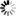Effat University Library Catalog
Normal view

# Beginning and intermediate algebra /

Additional authors: Hornsby, John. | McGinnis, Terry.
Edition statement:Edition 6. Published by : Pearson Education Limited, (New York :) Physical details: 1 volume (various pagings) : illustrations (some color) ; 29 cm ISBN:9780321969163.
Subject(s): Algebra -- Textbooks.
Year: 2016
Tags from this library: No tags from this library for this title.Average rating: 0.0 (0 votes)
Item type Current location Collection Call number Copy number Status Notes Date due Barcode
Textbook2 EFFAT UNIVERSITY LIBRARY
Reserve Collection
Non-fiction QA152.3 .L52 2016 (Browse shelf) c. 1 Available This textbook is allowed for 2-weeks loan only. a31111000038564

Includes index.

The real number system -- Linear equations and inequalities in one variable -- Linear equations in two variables -- Exponents and polynomials -- Factoring and applications -- Rational expressions and applications -- Graphs, linear equations, and functions -- Systems of linear equations -- Inequalities and absolute value -- Roots, radicals, and root functions -- Quadratic equations, inequalities, and functions -- Inverse, exponential, and logarithmic functions -- Nonlinear functions, conic sections, and nonlinear systems -- Sequences and series -- Appendices.

There are no comments for this item.